Science, Maths & Technology

### Become an OU student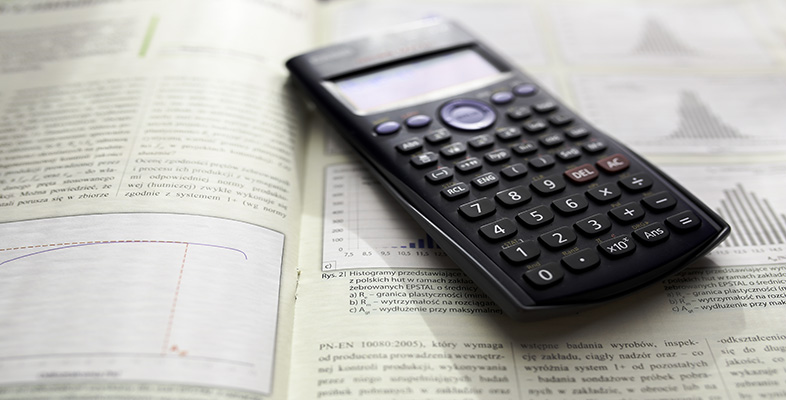Using a scientific calculator

Start this free course now. Just create an account and sign in. Enrol and complete the course for a free statement of participation or digital badge if available.

# 1 Getting to know your calculator

The first 11 sections describe how to use the calculator and how to perform different types of calculations. Section 12 contains a calculator reference guide that you can refer to as needed for some of the main key sequences.

This course is not an exhaustive list of all the calculator’s features. If you are using a different calculator, you should use the corresponding features of your own calculator to do the activities in this guide. You may need to refer to your calculator manual to do this.

The first step in using your calculator effectively is to make sure that you are familiar with the layout of the keys on the keypad, and that you can understand the information on the display.

Figure 1 shows the different parts of the Casio fx-83ES calculator.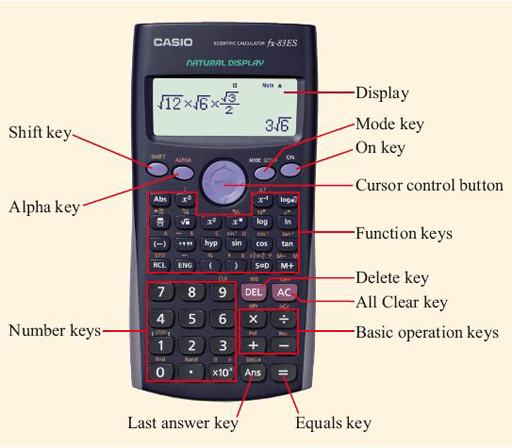Figure 1 A typical scientific calculator

If you are using a different model of calculator, make sure that you can identify similar functions on your model.

The calculator is switched on using the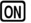key at the top right-hand corner of the keypad. Figure 2 shows the different elements of this calculator’s display.

Throughout this section, calculator keys will be indicated using the symbol on the key enclosed in a box, for example.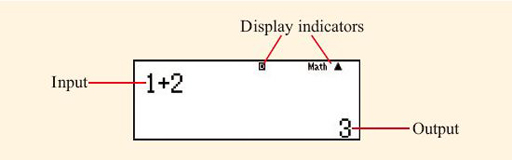Figure 2 The calculator display

The lower half of the keypad contains the number keys, keys for the basic operations of addition, subtraction, division and multiplication, and the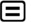key, which is pressed when you want the calculator to display the result of the calculation you have entered. The keys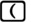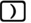used to insert brackets into a calculation are in the centre of the row above the number keys.

Many keys on the calculator have more than one use. The main function of a key is printed in white on the key itself. The second function of the key is printed in yellow above the key, and is accessed by pressing the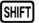button before pressing the key. When you press thebutton, the symbol ‘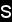’ appears at the top left-hand corner of the calculator display to remind you that the button has been pressed. It disappears when you press another key. Some keys also have a third function, printed above the key in red. These functions allow numerical values stored in the calculator memories to be used within calculations and are accessed by pressing the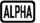button before the appropriate key. When thebutton is pressed, the symbol ‘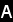’ is shown at the top of the calculator display. You will learn how to use the calculator memories later in section 4.

The calculator manual describes this second function as the ‘alternate’ function of the key.

Some calculator operations are accessed through a system of menus that are displayed on the calculator screen, as shown in Figure 3. The required menu option is selected by pressing the number key associated with the option, as given on the calculator screen.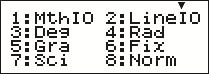Figure 3 A typical calculator on-screen menu

When describing how to use various calculator functions, this guide gives the exact keys that you need to press using the symbols shown on the keys. This is known as a ‘key sequence’. If the key sequence accesses the second function of a key, or a function from a menu, the name of this function will be given in brackets at the appropriate point in the key sequence. Names in brackets are thus not keys that you press but simply describe the function that is accessed using the previous key sequence. For example, to turn off the calculator, press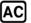(OFF). In this notation, (OFF) is not a key that you press, but is the name of the second function of thekey, which is accessed with thekey.

The calculator has many modes of operation that affect how mathematics is entered and displayed. These will be described later in this guide, but before progressing any further you should reset your calculator to the default course settings.

## Activity 1 Initialising your calculator

To initialise your calculator to the default course settings, turn it on and then enter the following two key sequences: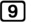(CLR)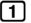(Setup)(Yes)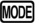(SETUP)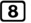(Norm)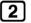Note that in the first key sequence, ‘CLR’ (which is short for ‘clear’) is the second function of thekey, and ‘Setup’ is the name of the on-screen menu option corresponding to thekey. ‘Yes’ is the name of the on-screen menu option corresponding to thekey. This key sequence clears all previous ‘setup’ settings on the calculator.

In the second key sequence, ‘SETUP’ is the name of the second function of thekey, and ‘Norm’ (short for ‘normal’) is the on-screen menu option corresponding to thekey. Pressing the keyselects to use ‘Normal 2’ mode, which will be described in more detail in section 5.

Note the difference between ‘SETUP’, the second function of thekey, and the menu option ‘Setup’.

Your calculator will now be working in ‘Math’ mode, and the word Math will be shown near the right-hand side of the top of the calculator display, as shown in Figure 4 below. Math mode is the recommended way of using your calculator during this course as it allows mathematics to be entered and displayed in a similar way to how you would write it on paper.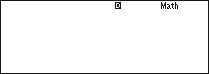Figure 4 ‘Math’ mode